# AccelerationPage 1

#### WATCH ALL SLIDES

Slide 1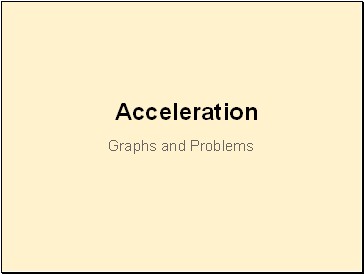## Acceleration

Graphs and Problems

Slide 2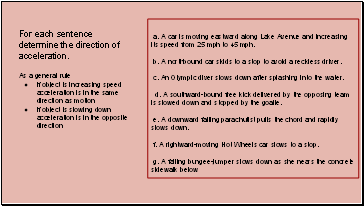a. A car is moving eastward along Lake Avenue and increasing its speed from 25 mph to 45 mph.

b. A northbound car skids to a stop to avoid a reckless driver.

c. An Olympic diver slows down after splashing into the water.

d. A southward-bound free kick delivered by the opposing team is slowed down and stopped by the goalie.

e. A downward falling parachutist pulls the chord and rapidly slows down.

f. A rightward-moving Hot Wheels car slows to a stop.

g. A falling bungee-jumper slows down as she nears the concrete sidewalk below

For each sentence determine the direction of acceleration.

As a general rule

if object is increasing speed acceleration is in the same direction as motion

if object is slowing down acceleration is in the opposite direction

Slide 3## Representing acceleration graphically

Describe the motion indicated by the graphs.

Slide 4## Examples - calculating Acceleration

The velocity of the aircraft is reduced from 100 m/s[S] to 40 m/s[S] in 8 s. Find it’s average acceleration.

Slide 5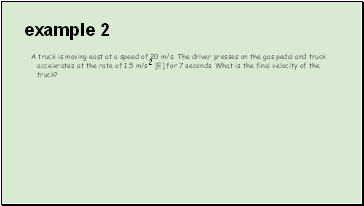example 2

A truck is moving east at a speed of 20 m/s. The driver presses on the gas pedal and truck accelerates at the rate of 1.5 m/s2 [E] for 7 seconds. What is the final velocity of the truck?

Slide 6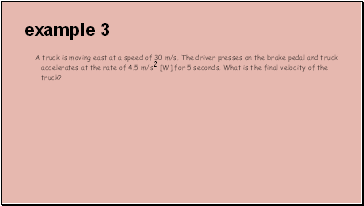example 3

A truck is moving east at a speed of 30 m/s. The driver presses on the brake pedal and truck accelerates at the rate of 4.5 m/s2 [W] for 5 seconds. What is the final velocity of the truck?

Slide 7Kinematic Equations - found in Motion text section 2.5

, always true

, always true

, constant acceleration only

, constant acceleration only

, constant acceleration only

, constant acceleration only

d

d

Slide 8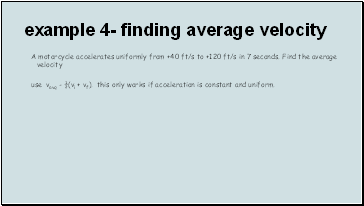example 4- finding average velocity

A motorcycle accelerates uniformly from +40 ft/s to +120 ft/s in 7 seconds. Find the average velocity

use vavg - ½(vi + vf). this only works if acceleration is constant and uniform.

Slide 9example 5

A car is moving at +25 m/s. It then accelerates at a rate of 1.5 m/s2 for 10 s. Find it’s final velocity.

Go to page:
1  2